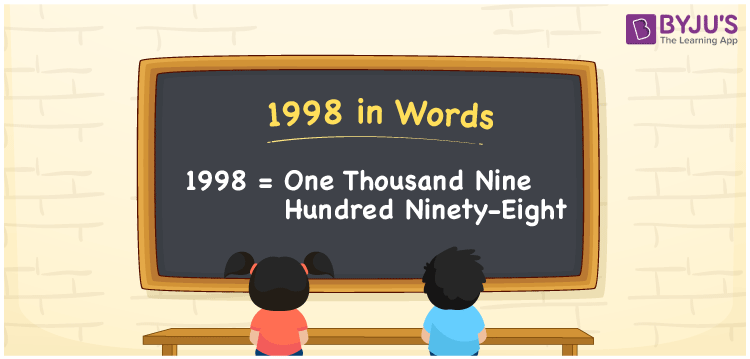# 1998 in words

1998 in words is written as One Thousand Nine Hundred and Ninety Eight. 1998 represents the count or value. The article on Counting Numbers can give you an idea about count or counting. The number 1998 is used in expressions that relate to money, distance, length, year and others. Let us consider an example for 1998. “Beena was born in the year 1998”. Another example, “There are One thousand Nine Hundred and Ninety Eight pages in a Novel.”

 1998 in words One Thousand Nine Hundred and Ninety Eight One Thousand Nine Hundred and Ninety Eight in Numbers 1998

## 1998 in English Words## How to Write 1998 in Words?

We can convert 1998 to words using a place value chart. This can be done as follows. The number 1998 has 4 digits, so let’s make a chart that shows the place value up to 4 digits.

 Thousands Hundreds Tens Ones 1 9 9 8

Thus, we can write the expanded form as:

1 × Thousand + 9 × Hundred + 9 × Ten + 8 × One

= 1 × 1000 + 9 × 100 + 9 × 10 + 8 × 1

= 1998

= One Thousand Nine Hundred and Ninety Eight.

1998 is the natural number that is succeeded by 1997 and preceded by 1999.

1998 in words – One Thousand Nine Hundred and Ninety Eight.

Is 1998 an odd number? – No.

Is 1998 an even number? – Yes.

Is 1998 a perfect square number? – No.

Is 1998 a perfect cube number? – No.

Is 1998 a prime number? – No.

Is 1998 a composite number? – Yes

## Solved Example

1. Write the number 1998 in expanded form

Solution: 1 × 1000 + 9 × 100 + 9 × 10 + 8 × 1

We can write 1998 = 1000 + 900 + 90 + 8

= 1 × 1000 + 9 × 100 + 9 × 10 + 8 × 1

## Frequently Asked Questions on 1998 in words

Q1

### How to write 1998 in words?

1998 in words is written as One Thousand Nine Hundred and Ninety Eight.
Q2

### State whether True or False. 1998 is divisible by 2?

True. 1998 is divisible by 2.
Q3

### Is 1998 divisible by 10?

No. 1998 is not divisible by 10.